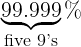# Converting between nines and sigmas

Nines and sigmas are two ways to measure quality. You’ll hear something has four or five nines of reliability or that some failure is a five sigma event. What do these mean, and how do you convert between them?

## Definitions

If a system has fives nines of availability, that means the probability of the system being up is 99.999% Equivalently, the probability of it being down is 0.00001.In general, n nines of availability means the probability of failure is 10n.

If a system has s sigmas of reliability, that means the probability of failure is the same as the probability of a Gaussian random variable being s standard deviations above its mean .

## Conversion formulas

Let Φ be the cumulative density function for a standard normal, i.e. a Gaussian random variable with mean zero and standard deviation 1. Then s sigmas corresponds to n nines, where

n = -log10(Φ(-s))

and

s = -Φ-1(10n).

We’ll give approximate formulas in just a second that don’t involve Φ but just use functions on a basic calculator.

Here’s a plot showing the relationship between nines and sigmas.The plot looks a lot like a quadratic, and in fact if we take the square root we get a plot that’s visually indistinguishable from a straight line. This leads to very good approximations

n ≈ (0.47 + 0.42 s

and

s ≈ 2.37 √n – 1.12.

The approximation is so good that it’s hard to see the difference between it and the exact value in a plot.These approximations are more than adequate since nines and sigmas are crude measures, not accurate to more than one significant figure .

## Related posts

 Here I’m considering the one-tailed case, the probability of being so many standard deviations above the mean. You could consider the two-tailed version, where you look at the probability of being so many standard deviations above or below the mean. The two-tailed probability is simply twice the one-tailed probability by symmetry.

 As I’ve written elsewhere, I’m skeptical of the implicit normal distribution assumption, particularly for rare events. The normal distribution is often a good modeling assumption in the middle, but not so often in the tails. Going out as far as six sigmas is dubious, and so the plot above covers as much range as is practical and then some.

## 2 thoughts on “Converting between nines and sigmas”

1. Steve Black

… IF (big if) the distribution is Gaussian.